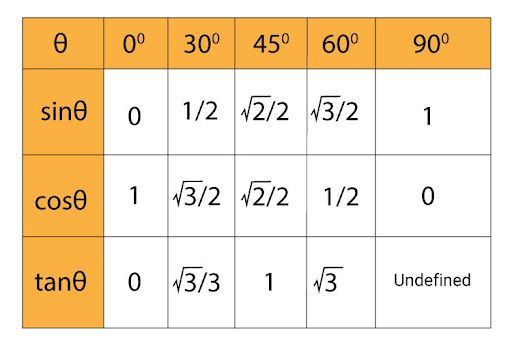# Show that the value of cos(30) x tan(60) + sin(30) is an integerIn case the question states that you are not allowed to use the calculator, then you are supposed to remember the values given for each trigonometric ratio in the table above. If it allows you to use the calculator, then you can just directly find the values of each ratio, substitute them in the equation and solve for the answer.

As we know that:

cos 30= (√3)/2

tan 60= √3

sin 30=½

So we just substitute these values in the above-given equation

=(√3)/2 × √3 + ½

=(√3)2୵2 + ½

= 3/2 + ½

=4/2

=2